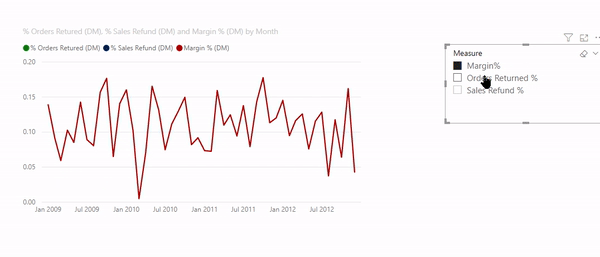top of page
Search

# Show or Hide measures using Slicer: Power BIUsing slicers, we can control the measure to display in a visual:Unlike the method explained in the previous article, this method enables multiple selections of measures.Let us see how to set this up.

## Step 1: Creating a table for slicer

Like the previous article, we add a disconnected table in the model with the list of all the required measuresThe name of the table in this example is Measure Selection Table

## Step 2: Add a slicer on the page

Add a slicer on the page and use the column Measure from the table created in the previous step:## Step 3: Modify the existing measures

Original measures:

``` % Orders Returned =
DIVIDE([Returned Orders],[Total Orders])

% Sales Refund =
DIVIDE([Returned Sales Amount],[Sales Amount])
```

For this exercise, we need to modify the above measures using IF and CONTAINS

``` % Orders Returned =
IF(
CONTAINS('Measure Selection Table','Measure Selection Table'[Index],2),
DIVIDE([Returned Orders],[Total Orders])
)
```

In simple English, we are telling Power BI to run the calculation of % Order Returns, IF the slicer selection is Order Returned (corresponding Index row value = 2).

### CONTAINS

CONTAINS DAX returns true if values for all referred columns exist, or are contained, in those columns; otherwise, the function returns false.

#### SyntaxIt has 3 required arguments: Table name, Column name, and the corresponding value.

For example, in the following formula, we are asking DAX to check in the table Measure Selection Table check for value 2 in the Index column.

`CONTAINS('Measure Selection Table’, ‘Measure Selection Table'[Index],2)`

Modify the other measures accordingly:

``` % Sales Refund =
IF(
CONTAINS('Measure Selection Table','Measure Selection    Table'[Index],3),
DIVIDE([Returned Sales Amount],[Sales Amount])
)

Margin % =
VAR _SalesAmt =
SUMX(dtOrders,dtOrders[Unit Price] * dtOrders[Order Quantity])

VAR _TotalCost =
SUMX(dtOrders,dtOrders[Unit Cost] * dtOrders[Order Quantity])

VAR _Margin =
_SalesAmt - _TotalCost

VAR _MarginPrcnt =
DIVIDE(_Margin, _TotalCost)

VAR _Show =
IF(
CONTAINS('Measure Selection Table','Measure Selection Table'[Index],1),
_MarginPrcnt
)

RETURN
_Show
```

## Step 4: Use the above-created measures in the visualAnd that is it!